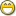### Re: Wrap-around search algorithm

Pseudo code:

This way you can even highlight all the matches, but make the current one a bright highlight:

```sequence matches
sequence lastStrFind = ""

procedure find(sequence strFind = lastStrFind, integer bDirection)
integer cursorPos = current_cursor_position()

if length(strFind) = 0 then
if length(lastStrFind) = 0 then
return
else
strFind = lastStrFind
end if
end if

matches = match_all( strFind, docText )

if bDirection = Forward then
if cursorPos < matches then
-- at the top, just go to first found

elsif cursorPos > matches[\$] then
-- at end of document, wrap to first in matches[]

else
for t=1 to length(matches) docText
if matches[t] > cursorPos then
highlightFound( matches[t-1], length(strFind) )
exit
end if
end for
end if
else
-- do it backwards style
end if

lastStrFind = strFind
end procedure
```

This way you don't have to keep the matching string in memory, but it doesn't allow you to highlight all the matches:

```pos = match( strFind, docText, currentCursorPosition )

if pos = 0 then
pos = match( strFind, docText, 0 )
if pos = 0 then
end if
else
setHighlight( pos, length(strFind) )
end if
```

I also like in MS VS Code how if I select text, it lightly highlights all matching text in the document. If I highlight a variable name, for example, it subtly shows me all places on the page where that variable appears. Very nice!

Looks like Notepad++ also does the selection highlighting.